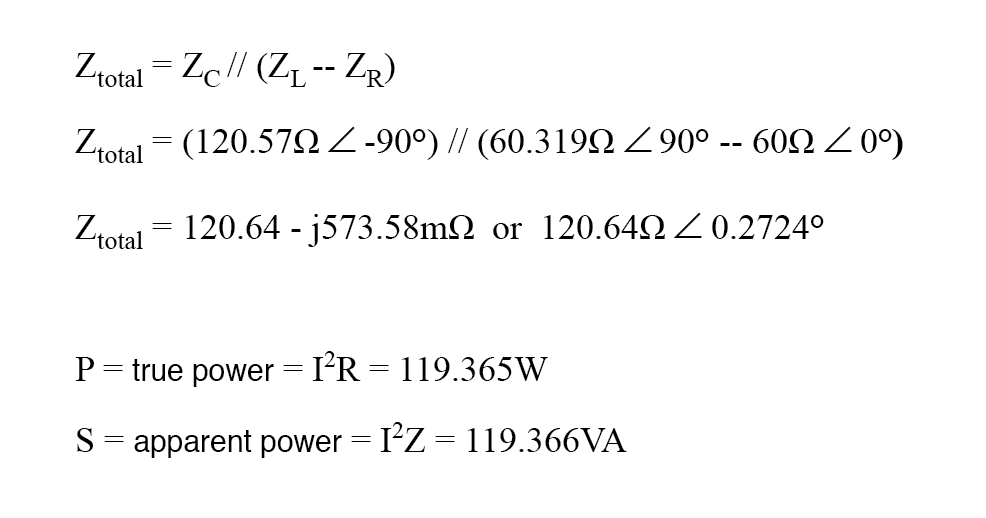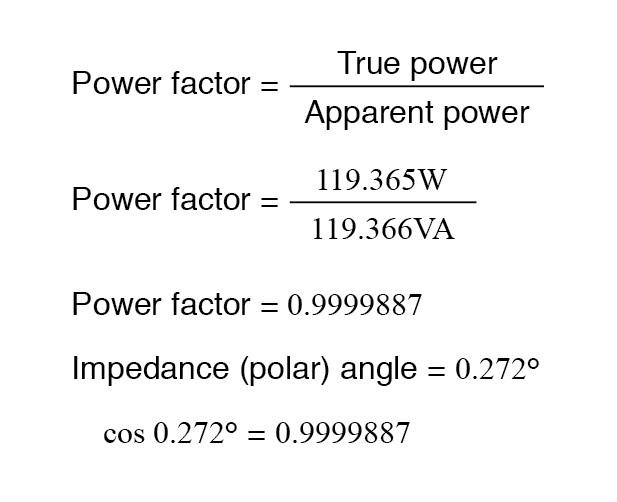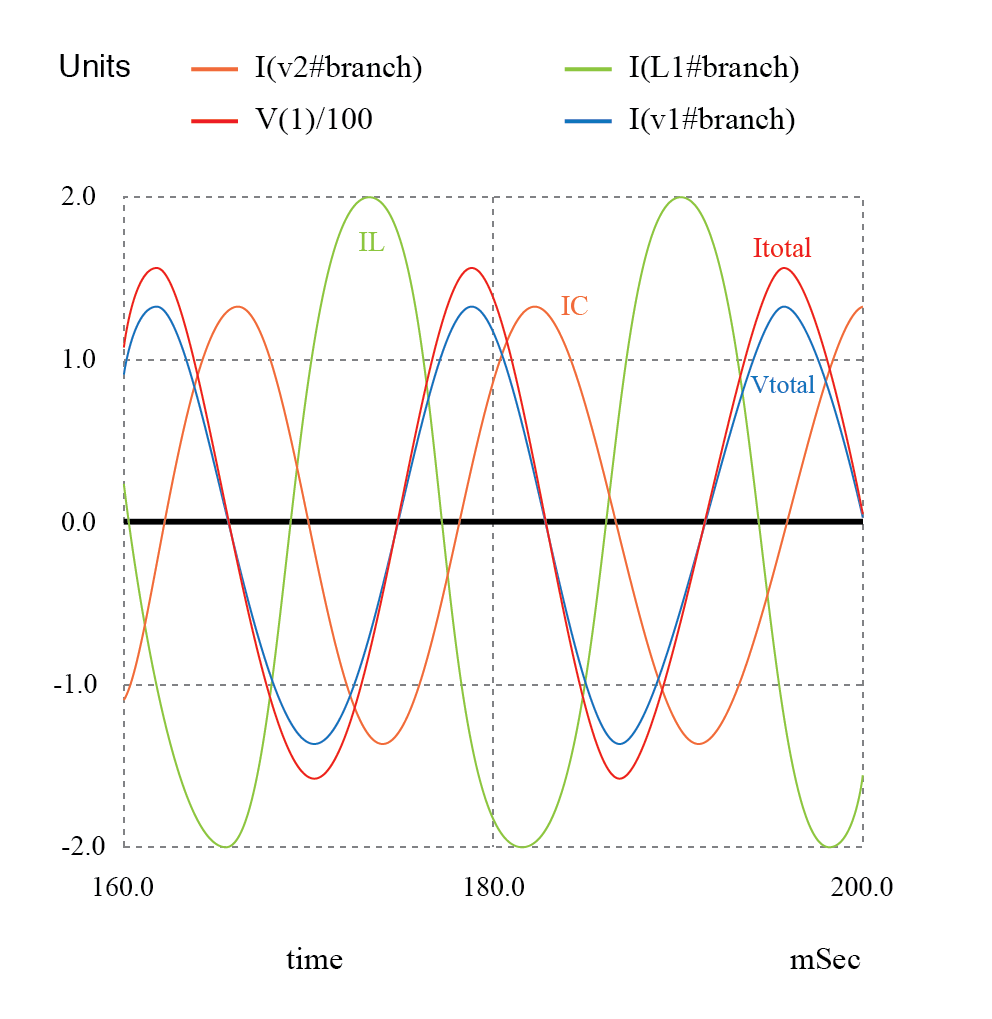# Calculating Power Factor

Thursday - 05/09/2019 14:50
As was mentioned before, the angle of this “power triangle” graphically indicates the ratio between the amount of dissipated (or consumed) power and the amount of absorbed/returned power.
It also happens to be the same angle as that of the circuit’s impedance in polar form. When expressed as a fraction, this ratio between true power and apparent power is called the power factor for this circuit.

Because true power and apparent power form the adjacent and hypotenuse sides of a right triangle, respectively, the power factor ratio is also equal to the cosine of that phase angle. Using values from the last example circuit:It should be noted that power factor, like all ratio measurements, is a unitless quantity.

Power Factor Values

For the purely resistive circuit, the power factor is 1 (perfect), because the reactive power equals zero. Here, the power triangle would look like a horizontal line, because the opposite (reactive power) side would have zero length.

For the purely inductive circuit, the power factor is zero, because true power equals zero. Here, the power triangle would look like a vertical line, because the adjacent (true power) side would have zero length.

The same could be said for a purely capacitive circuit. If there are no dissipative (resistive) components in the circuit, then the true power must be equal to zero, making any power in the circuit purely reactive. The power triangle for a purely capacitive circuit would again be a vertical line (pointing down instead of up as it was for the purely inductive circuit).

Importance of Power Factor

Power factor can be an important aspect to consider in an AC circuit because of any power factor less than 1 means that the circuit’s wiring has to carry more current than what would be necessary with zero reactance in the circuit to deliver the same amount of (true) power to the resistive load.

If our last example circuit had been purely resistive, we would have been able to deliver a full 169.256 watts to the load with the same 1.410 amps of current, rather than the mere 119.365 watts that it is presently dissipating with that same current quantity. The poor power factor makes for an inefficient power delivery system.

Poor Power Factor

Poor power factor can be corrected, paradoxically, by adding another load to the circuit drawing an equal and opposite amount of reactive power, to cancel out the effects of the load’s inductive reactance. Inductive reactance can only be canceled by capacitive reactance, so we have to add a capacitor in parallel to our example circuit as the additional load. The effect of these two opposing reactances in parallel is to bring the circuit’s total impedance equal to its total resistance (to make the impedance phase angle equal, or at least closer, to zero).

Since we know that the (uncorrected) reactive power is 119.998 VAR (inductive), we need to calculate the correct capacitor size to produce the same quantity of (capacitive) reactive power. Since this capacitor will be directly in parallel with the source (of known voltage), we’ll use the power formula which starts from voltage and reactance:Let’s use a rounded capacitor value of 22 µF and see what happens to our circuit: (Figure below)The power factor for the circuit, overall, has been substantially improved. The main current has been decreased from 1.41 amps to 994.7 milliamps, while the power dissipated at the load resistor remains unchanged at 119.365 watts. The power factor is much closer to being 1:Since the impedance angle is still a positive number, we know that the circuit, overall, is still more inductive than it is capacitive. If our power factor correction efforts had been perfectly on-target, we would have arrived at an impedance angle of exactly zero, or purely resistive. If we had added too large of a capacitor in parallel, we would have ended up with an impedance angle that was negative, indicating that the circuit was more capacitive than inductive.

A SPICE simulation of the circuit of (Figure above) shows total voltage and total current are nearly in phase. The SPICE circuit file has a zero volt voltage source (V2) in series with the capacitor so that the capacitor current may be measured. The start time of 200 msec ( instead of 0) in the transient analysis statement allows the DC conditions to stabilize before collecting data. See SPICE listing “pf.cir power factor”.

Pf
.cir
power factor V1 1 0 sin(0 170 60)
C1 1 3 22uF v2 3 0 0
L1 1 2 160mH
R1 2 0 60
# resolution stop start
.tran 1m 200m 160m
.end

The Nutmeg plot of the various currents with respect to the applied voltage Vtotal is shown in (Figure below). The reference is Vtotal, to which all other measurements are compared. This is because the applied voltage, Vtotal, appears across the parallel branches of the circuit. There is no single current common to all components. We can compare those currents to Vtotal.Note that the total current (Itotal) is in phase with the applied voltage (Vtotal), indicating a phase angle of near zero. This is no coincidence. Note that the lagging current, IL of the inductor would have caused the total current to have a lagging phase somewhere between (Itotal) and IL. However, the leading capacitor current, IC, compensates for the lagging inductor current. The result is a total current phase-angle somewhere between the inductor and capacitor currents. Moreover, that total current (Itotal) was forced to be in-phase with the total applied voltage (Vtotal), by the calculation of an appropriate capacitor value.

Since the total voltage and current are in phase, the product of these two waveforms, power, will always be positive throughout a 60 Hz cycle, real power as in the figure above. Had the phase-angle not been corrected to zero (PF=1), the product would have been negative where positive portions of one waveform overlapped negative portions of the other as in the figure above. Negative power is fed back to the generator. It cannot be sold; though, it does waste power in the resistance of electric lines between load and generator. The parallel capacitor corrects this problem.

Note that the reduction of line losses applies to the lines from the generator to the point where the power factor correction capacitor is applied. In other words, there is still circulating current between the capacitor and the inductive load. This is not normally a problem because the power factor correction is applied close to the offending load, like an induction motor.

It should be noted that too much capacitance in an AC circuit will result in a low power factor just as well as too much inductance. You must be careful not to over-correct when adding capacitance to an AC circuit. You must also be very careful to use the proper capacitors for the job (rated adequately for power system voltages and the occasional voltage spike from lightning strikes, for continuous AC service, and capable of handling the expected levels of current).

If a circuit is predominantly inductive, we say that its power factor is lagging (because the current wave for the circuit lags behind the applied voltage wave). Conversely, if a circuit is predominantly capacitive, we say that its power factor is leading. Thus, our example circuit started out with a power factor of 0.705 lagging and was corrected to a power factor of 0.999 lagging.
Key: Power